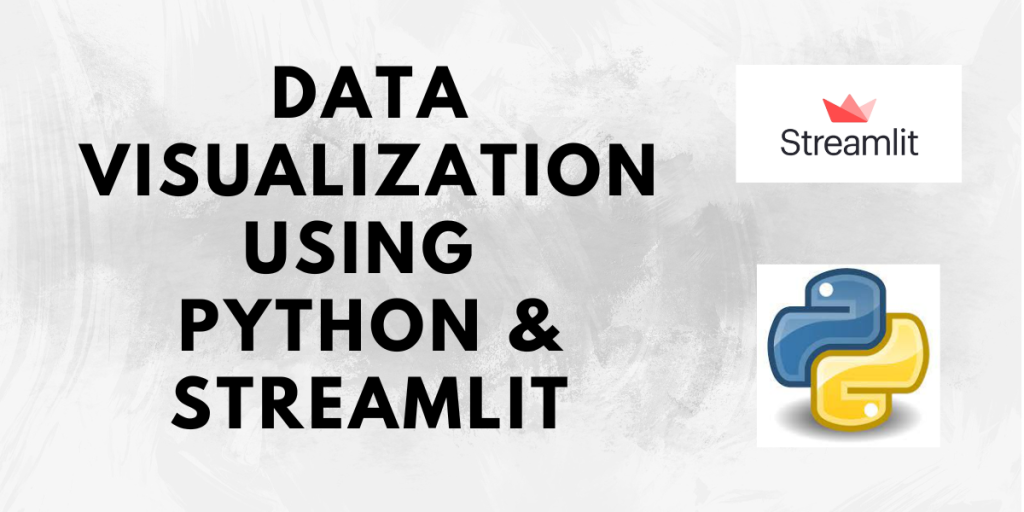# Data Visualization using Streamlit – A Complete GuideData visualisation is the representation of data in a graphical format. It helps us to understand the data better and in an easier way. Data visualisation can be performed with the help of visual elements like charts, graphs, maps, etc.

In this tutorial, we will learn how to plot different charts using Streamlit. So let us begin!

Also read: An Introduction to Streamlit

## Line Chart in Streamlit

It is a graphical representation of information performed by connecting that connects a series of data points as a continuous line. It is one of the simplest forms of data visualization techniques.

```import streamlit as st
import pandas as pd

data = {"a":[23, 12, 78, 4, 54], "b":[0, 13 ,88, 1, 3],
"c":[45, 2, 546, 67, 56]}

df = pd.DataFrame(data)
df
st.line_chart(data=df)

```

Output:

Here, we have taken some arbitrary data and created a data frame first using the Pandas library and then plotted the chart using the `line_chart` function. We have also displayed the data frame before plotting the graph for quick reference.

Also read: Calculator App using Streamlit – Easy Step-by-Step Method

## Bar Chart in Streamlit

A bar chart represents data in the form of vertical or horizontal rectangular bars. The height of each bar is proportional to the value that it represents. Streamlit provide a `bar_chart` function to plot bar charts for data visualization.

```import streamlit as st
import pandas as pd

data = {"a":[23, 12, 78, 4, 54], "b":[0, 13 ,88, 1, 3],
"c":[45, 2, 546, 67, 56]}

df = pd.DataFrame(data)
df
st.bar_chart(data=df)

```

Output:

Since the data frame contains 5 rows, there are bars corresponding to each row in the output. These bars contain the distribution of each column’s value in that specific row and are denoted by different colours for each column.

Also read: Deploying a Machine Learning Model using Streamlit – House Price Prediction GUI

## Area Chart in Streamlit

An area chart is a combination of both a line chart and a bar chart. The data points are plotted and then connected by lines. Then, the area below the line is coloured. Similarly, the other values are also plotted and the area is then coloured resulting in a layered chart. We can plot such a chart using the `area_chart` function from Streamlit.

```import streamlit as st
import pandas as pd

data = {"a":[23, 12, 78, 4, 54], "b":[0, 13 ,88, 51, 3],
"c":[45, 2, 46, 67, 56]}

df = pd.DataFrame(data)
df
st.area_chart(df)

```

Output:

Here the area of each column in the data frame is represented by a different colour as can be seen in the figure above. Some of the areas overlap as well depending on the data provided.

Also read: Theming in Streamlit – 2 Methods to Customize The Look and Feel of Streamlit apps

## Maps in Streamlit

We can also display maps in a Streamlit web app as a part of data visualization. The `map` function in Streamlit helps you display a map with data points on it. We can specify the data using the column names “lat” or “latitude” to denote the latitude and “lon” or “longitude” to denote the longitude. It is necessary to add these columns in the data to be plotted to plot the map.

```import streamlit as st
import pandas as pd

data = {"lat":[28.704060, 28.459497, 13.082680, 17.385044, 23.0225],
"lon":[77.102493, 77.026634, 80.270721, 78.486671, 72.5714],

df = pd.DataFrame(data)
df
st.map(data=df)

```

Output: